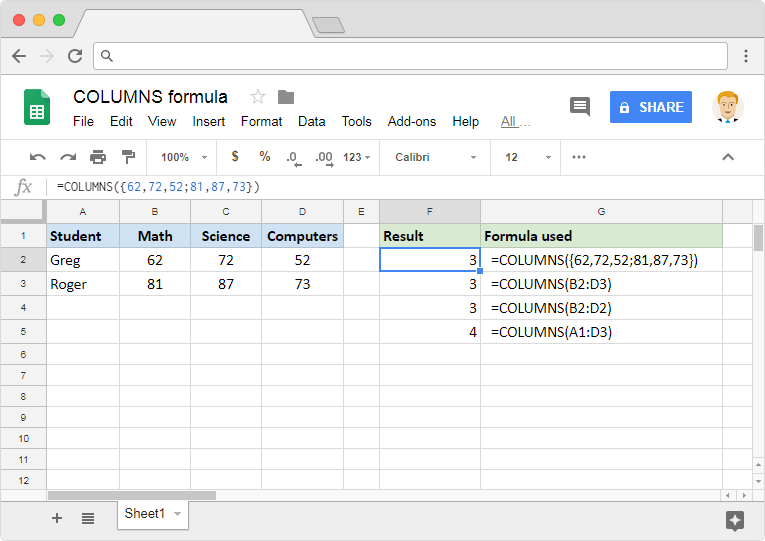# How to use the COLUMNS formula in Google SheetsLet us say we have a range of cells in a spreadsheet, and we want to know how many columns it has spread across. If there are only a few of them, we could probably do a quick observation and manually count the number. But there can be instances where columns in a range are more than just a few. Or we may need to generate the output dynamically, rather than relying upon manual observation. In such scenarios, the appropriately named COLUMNS formula in Google Sheets can help us.

### Syntax

COLUMNS(range)

• range – is the range of values, or the address reference to the range of cells, whose column count the formula returns.

### Usage: COLUMNS formula in Google Sheets

As such, the syntax of the formula is pretty straightforward. But going through a few practical examples reinforces our understanding. So, without further ado, let us dive right in. Please have a look at the snapshot below.In order for us to input values directly as a range, we need to use a pair of curly brackets. We illustrated this in the first example. How do we specify the columns and rows in such a range? It is simple, really. While we separate the values into columns using a comma, we use a semi-colon to separate the values into rows. And if we observe closely, the range {62,72,52;81,87,73} consists of two rows and three columns. Therefore the formula returned the output as 3.

In the second example, we used the reference to the range of cells instead of inputting the values directly. The range B2:D3 also spans across two rows and three columns. Hence it evaluates the output to 3, just like the formula in the first example did.

Regardless of the number of rows within the range, the COLUMNS formula returns only the number of columns within that range. The range B2:D2 used in the third example has just one row, but that doesn’t impact the output. That is because there are three columns from B through D.

The fourth example shows just another range, that consists of four columns. Hence the formula returns 4 as output.